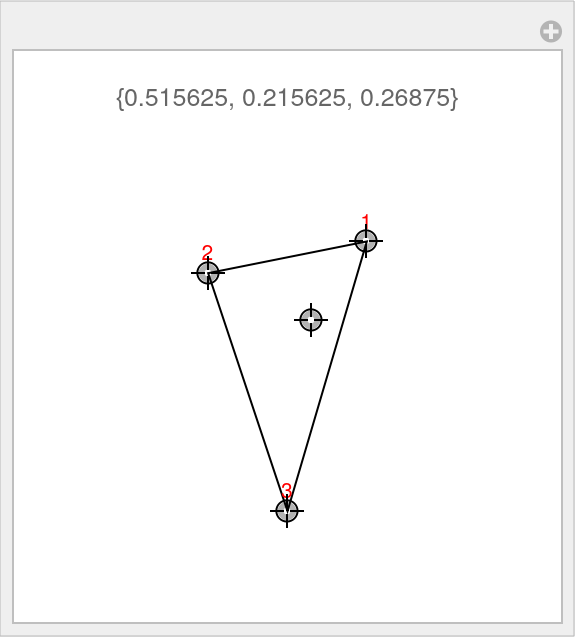Function Repository Resource:

BarycentricCoordinates

Find the barycentric coordinates of a point

Contributed by: Sander Huisman
 ResourceFunction["BarycentricCoordinates"][{p1,p2,…},p] finds the barycentric coordinates of point p in the coordinate system defined by the points pi.

Details and Options

For a point in n dimensions, the first argument should be n+1 points.
Find the values λ1, λ2, …, λn such that i=1nλipi=p and i=1nλi=1.
ResourceFunction["BarycentricCoordinates"] works in any dimension.

Examples

Basic Examples

Find the barycentric coordinates for the point {0.3,0.4} for the coordinate system {{1,1},{-1,1},{0,-1}}:

 In:=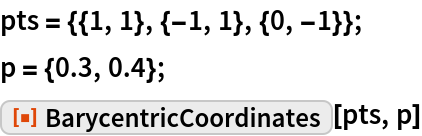Out=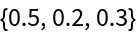Check the finding:

 In:=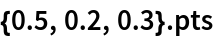Out=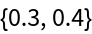Scope

Exact input leads to exact output:

 In:=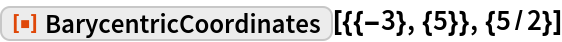Out=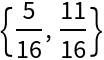BarycentricCoordinates works in 1D:

 In:=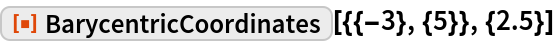Out=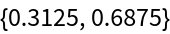BarycentricCoordinates also works in higher dimensions:

 In:=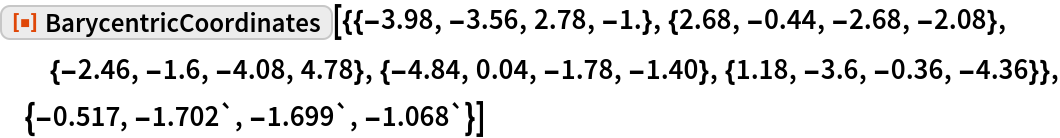Out=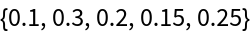BarycentricCoordinates also works in very high dimensions:

 In:=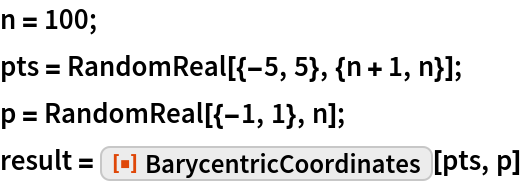Out=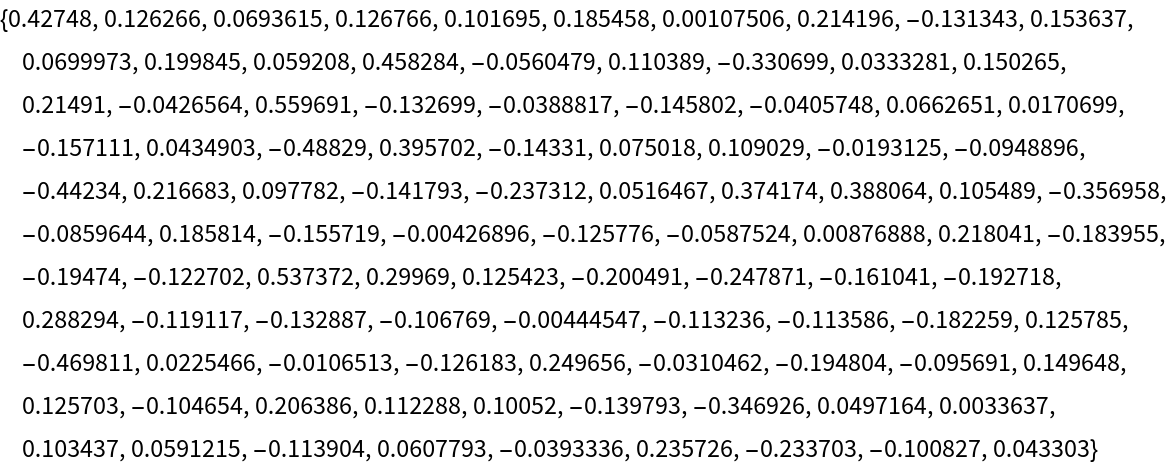Check that the barycentric coordinates add up to 1:

 In:=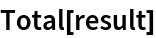Out=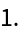Recreate the point p and subtract it from the original:

 In:=Out=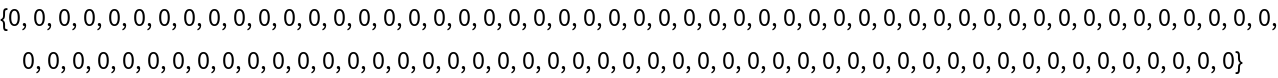Properties and Relations

The barycentric coordinates add up to 1:

 In:=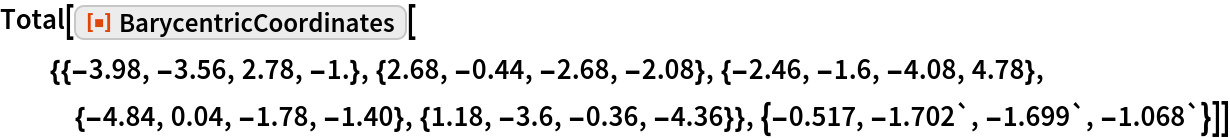Out=Possible Issues

Dimensions of the points have to agree with each other:

 In:=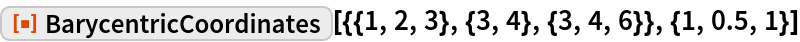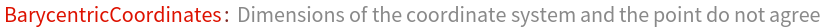Out=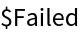Dimensions of the points have to agree with each other:

 In:=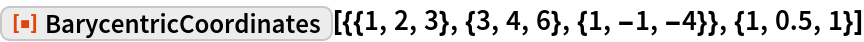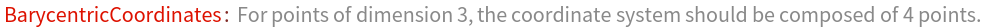Out=Neat Examples

Interactively move the points of the coordinate system:

 In:=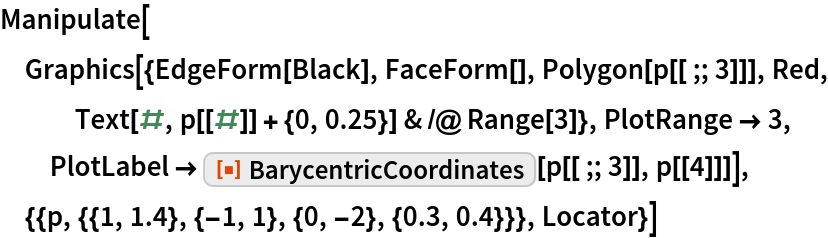Out=